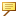Mass Property Functions
Mass Property Function
Description
massprop_param
Obtains the value of the mass property parameter.This function is applicable only when the mass properties source is defined as Fully Assigned.
Use the following relation to obtain the value of a mass property parameter:
"Paramname" = massprop_param(<"mass property name">)
where mass property name can be one of the following: AREA, VOLUME, DENSITY, XCG, YCG, ZCG, IXX, IYY, IZZ, IXY, IXZ, IYZ, INRT1, INRT2, and INRT3.
For example:
My_volume = massprop_param("VOLUME") — Obtains the value of the volume for the given model.
material_param
material_param (“PARAM_NAME”,“MATERIAL_NAME”)—Returns the value of “PARAM_NAME” from “MATERIAL_NAME” of the current model.
material_param (“PARAM_NAME”)—Returns the value of “PARAM_NAME” from the currently assigned material in the current model.
mp_mass
Returns the mass of a model part or assembly.
mp_mass (<"string">)
where string is an expression that is evaluated to string value.
For example:
mp_mass (“”) — returns mass of the given model.
mp_mass (“25:”) — returns mass of a component model (ID=25) that belongs to an assembly.
mp_mass(“25:32:”) — returns the mass of the component (ID=32) of a sub-assembly having component ID=25 that belongs to a top-level assembly.
mp_assigned_mass
Obtains mass of a model.
mp_assigned_mass (<"string">)This function is defined only when the mass properties of a model are assigned through a file.
mp_surf_area
Returns the area of a model part or assembly.
mp_surf_area (<"string">)
mp_volume
Returns the volume of a model part or assembly.
mp_volume (<"string">)
mp_cg_x
Obtains the X-coordinate of the center of gravity of the model.
mp_cg_x (<"string1">, <"coordinate system name">, <"string2">)
All 3 arguments are strings.
For example, mp_cg_x (“”, “my_csys”, “”) — obtains the X-coordinate of the given model in a coordinate system, named my_csys.
mp_cg_y
Obtains the Y-coordinate of the center of gravity of the model.
mp_cg_y (<"string1">, <"coordinate system name">, <"string2">)
mp_cg_z
Obtains the Z-coordinate of the center of gravity of the model.
mp_cg_z (<"string1">, <"coordinate system name">, <"string2">)